#The Wolfram Language:Fast Introduction for Programmers

All sections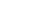Get Started

# ListsVideo Version

Lists are indicated in the Wolfram Language by {...}

Notes for Python programmers:

When coding in Python with the Wolfram Client Library for Python, classes like list, set and frozenset, as well as generator functions, are serialized as List.

They can contain any kinds of expressions:

In:=1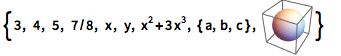`{3, 4, 5, 7/8, x, y, x^2 + 3 y^3, {a, b, c}, \!\(\*Graphics3DBox[SphereBox[{0, 0, 0}],ImageSize -> {46.62109375, Automatic},ViewPoint -> {1.3423934952878664`, -2.404165276254038,1.9667152890703756`},ViewVertical -> {0.009348068236588528, -0.008691644947737727, 0.9999185311455862}]\)}`

Notes for Java programmers:

In the Wolfram Language, lists represent grouped data. They can have any structure and size, and the language automatically infers the most efficient internal representation. Java programmers must decide between either primitive arrays or classes from the Collection Framework, and this choice is heavily dependent on context.

Parts of lists are indexed starting at 1, and can be extracted using [[ ... ]]

In:=2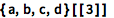`{a, b, c, d}[]`
Out=2Negative indices count from the end:

In:=3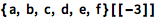`{a, b, c, d, e, f}[[-3]]`
Out=3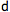Notes for Java programmers:

Like Java arrays, Wolfram Language lists are indicated by curly braces {...}. Indexing in the Wolfram Language uses [[...]] rather than [...], and indices start at 1 instead of 0.

Notes for Python programmers:

Wolfram Language lists are indicated by curly braces {...}, while Python lists use the same square bracket [...] syntax for both indicating lists and indexing them. The Wolfram Language avoids ambiguity by indexing with [[...]] and employing an intuitive indexing system that starts at 1 instead of 0.

Many operations immediately "thread" over lists:

In:=1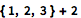`{1, 2, 3} + 2`
Out=1In:=2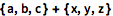`{a, b, c} + {x, y, z}`
Out=2Notes for Java programmers:

Threading operations over Java arrays or collections would require loop structures.

Notes for Python programmers:

In the Wolfram Language, + is strictly for arithmetic addition. String concatenation, for example, uses <>.

Refer to "spans" in lists using ;;

In:=1`{a, b, c, d, e, f}[[2 ;; 4]]`
Out=1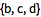Notes for Java programmers:

Java collections and arrays do not have anything comparable to Wolfram Language spans for extracting subsets.

Notes for Python programmers:

;; spans in the Wolfram Language are similar to the Python : slices, though they generalize to multidimensional arrays.

QUICK REFERENCE: Operations on Lists

Which of the following will get the third element of the list {x, y, z}?

Which of the following evaluates to 3?

Which of the following will get the element a from the list {a, b, c}?